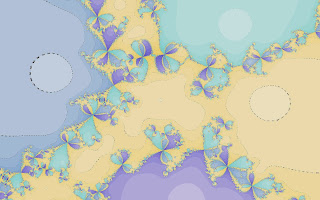## Monday, March 09, 2015

### Newton Method, Re-IteratedFigure 1: Cube Roots Of Unity, Rotated, Newton's Method

I have been re-visiting my program for drawing fractals with Newton's method. Newton's method is an iterative method for finding the roots of non-linear systems of equations. That is, it is used to find zeros of functions. For my purposes, Newton's method can be used to draw fractals, although I was pleased to learn a bit more about methods in numerical analysis. I made various improvements to my program, including the the implementation of:

• More polynomial functions whose zeros are desired.
• Rotations and reflections.
• Two additional iterative methods for root finding.

I was pleased that I had thought to define a Java interface for functions whose zeros were sought. (When one looks at one's own code from a couple years ago, one might as well as be looking at code by somebody else.) Each new function could be added by defining a class implementing this interface. Besides specific functions, I defined a general polynomial, with complex coefficients, that maps complex numbers into complex numbers. I defined rotations and reflections by the transformations to the zeros of this general polynomial. A different strategy would need to be specified if one wanted to create a program for drawing fractals for functions that are not limited to being polynomials.

Halley's method is derived from a second-order Taylor approximation. (Newton's method is derived from a first order approximation.) As nearly, as I can see, Halley's method does not produce as interesting fractals. In implementing the method, I had to review a bit about tensors, since the second derivative of a function mapping the real plane into the real plane is a tensor.Figure 2: Cube Roots Of Unity, Rotated, Halley's Method

I do not have much of an understanding of the rationale for the Chun-Neta method. I can see that it takes less iterations than Newton or Halley's method, although more calculations per iteration than either of those two methods. (The visual result of less iterations is a lighter color around the roots in the image below, as compared with above.) As I understand it, the black lines in the figure are an artifact of my implementation, probably resulting from dividing by zero.Figure 3: Cube Roots Of Unity, Rotated, Chun-Neta Method

I conclude with an example from a general polynomial, where I defined roots so that the resulting figures would have no obvious symmetries.Figure 4: A Fourth Degree Polynomial, Halley's MethodFigure 5: A Fourth Degree Polynomial, Chun-Neta Method
References
• Chun, C. and B. Neta (2011). A new sixth-order scheme for nonlinear equations. Applied Mathematics Letters.
• Scott, Melvin, B. Neta, and C. Chun (2011). Basin attractors for various methods. Applied Mathematics and Computation, V. 218: pp. 2584-2599.
• Yau, Lily and A. Ben-Israel (1998). The Newton and Halley methods for complex roots. American Mathematical Monthly, V. 105: pp. 806-818